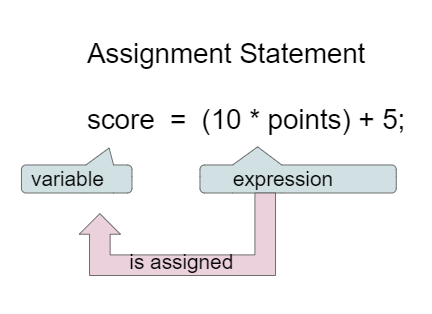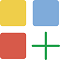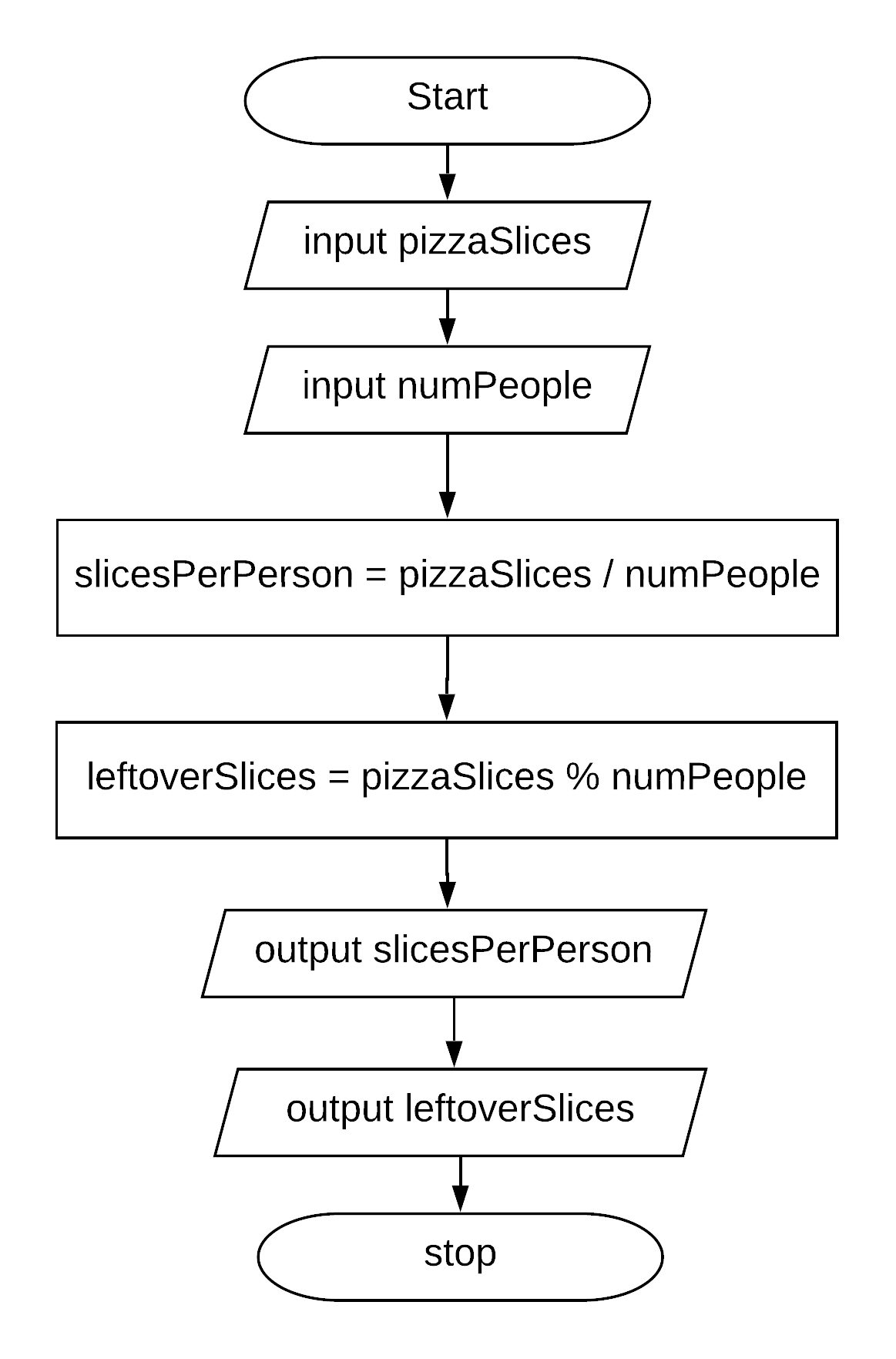# 1.4. Expressions and Assignment Statements¶

In this lesson, you will learn about assignment statements and expressions that contain math operators and variables.

## 1.4.1. Assignment Statements¶

Remember that a variable holds a value that can change or vary. Assignment statements initialize or change the value stored in a variable using the assignment operator `=`. An assignment statement always has a single variable on the left hand side of the = sign. The value of the expression on the right hand side of the = sign (which can contain math operators and other variables) is copied into the memory location of the variable on the left hand side.Figure 1: Assignment Statement (variable = expression)

Instead of saying equals for the `=` operator in an assignment statement, say “gets” or “is assigned” to remember that the variable on the left hand side gets or is assigned the value on the right. In the figure above, score is assigned the value of 10 times points (which is another variable) plus 5.

The following video by Dr. Colleen Lewis shows how variables can change values in memory using assignment statements.

As we saw in the video, we can set one variable to a copy of the value of another variable like y = x;. This won’t change the value of the variable that you are copying from.Coding Exercise:

Click on the `Show CodeLens` button to step through the code and see how the values of the variables change.

The program is supposed to figure out the total money value given the number of dimes, quarters and nickels. There is an error in the calculation of the total. Fix the error to compute the correct amount.

Calculate and print the total pay given the weekly salary and the number of weeks worked. Use string concatenation with the totalPay variable to produce the output `Total Pay = \$3000`. Don’t hardcode the number 3000 in your print statement.Check your understandingCoding Exercise:

Assume you have a package with a given height 3 inches and width 5 inches. If the package is rotated 90 degrees, you should swap the values for the height and width. The code below makes an attempt to swap the values stored in two variables h and w, which represent height and width. Variable h should end up with w’s initial value of 5 and w should get h’s initial value of 3. Unfortunately this code has an error and does not work. Use the CodeLens to step through the code to understand why it fails to swap the values in h and w.

1-4-7: Explain in your own words why the `ErrorSwap` program code does not swap the values stored in h and w.Check your understanding

Swapping two variables requires a third variable. Before assigning `h = w`, you need to store the original value of `h` in the temporary variable. In the mixed up programs below, drag the blocks to the right to put them in the right order.

The following has the correct code that uses a third variable named “temp” to swap the values in h and w.

The code is mixed up and contains <b>one extra block</b> which is not needed in a correct solution. Drag the needed blocks from the left into the correct order on the right, then check your solution. You will be told if any of the blocks are in the wrong order or if you need to remove one or more blocks.

After three incorrect attempts you will be able to use the <i>Help Me</i> button to make the problem easier.

Fix the code below to perform a correct swap of h and w. You need to add a new variable named `temp` to use for the swap.

## 1.4.2. Incrementing the value of a variable¶

If you use a variable to keep score you would probably increment it (add one to the current value) whenever score should go up. You can do this by setting the variable to the current value of the variable plus one (score = score + 1) as shown below. The formula looks a little crazy in math class, but it makes sense in coding because the variable on the left is set to the value of the arithmetic expression on the right. So, the score variable is set to the previous value of score + 1.Coding Exercise:

Click on the `Show CodeLens` button to step through the code and see how the score value changes.Check your understanding

## 1.4.3. Operators¶

Java uses the standard mathematical operators for addition (`+`), subtraction (`-`), multiplication (`*`), and division (`/`). Arithmetic expressions can be of type int or double. An arithmetic operation that uses two int values will evaluate to an int value. An arithmetic operation that uses at least one double value will evaluate to a double value. (You may have noticed that + was also used to put text together in the input program above – more on this when we talk about strings.)

Java uses the operator `==` to test if the value on the left is equal to the value on the right and `!=` to test if two items are not equal. Don’t get one equal sign `=` confused with two equal signs `==`! They mean different things in Java. One equal sign is used to assign a value to a variable. Two equal signs are used to test a variable to see if it is a certain value and that returns true or false as you’ll see below. Use == and != only with int values and not doubles because double values are an approximation and 3.3333 will not equal 3.3334 even though they are very close.Coding Exercise:

Run the code below to see all the operators in action. Do all of those operators do what you expected? What about `2 / 3`? Isn’t surprising that it prints `0`? See the note below.

Note

When Java sees you doing integer division (or any operation with integers) it assumes you want an integer result so it throws away anything after the decimal point in the answer, essentially rounding down the answer to a whole number. If you need a double answer, you should make at least one of the values in the expression a double like 2.0.

With division, another thing to watch out for is dividing by 0. An attempt to divide an integer by zero will result in an ArithmeticException error message. Try it in one of the active code windows above.

Operators can be used to create compound expressions with more than one operator. You can either use a literal value which is a fixed value like 2, or variables in them. When compound expressions are evaluated, operator precedence rules are used, so that *, /, and % are done before + and -. However, anything in parentheses is done first. It doesn’t hurt to put in extra parentheses if you are unsure as to what will be done first.Coding Exercise:

In the example below, try to guess what it will print out and then run it to see if you are right. Remember to consider operator precedence.Check Your Understanding

## 1.4.4. The Modulo Operator¶

The percent sign operator (`%`) is the mod (modulo) or remainder operator. The mod operator (`x % y`) returns the remainder after you divide `x` (first number) by `y` (second number) so `5 % 2` will return 1 since 2 goes into 5 two times with a remainder of 1. Remember long division when you had to specify how many times one number went into another evenly and the remainder? That remainder is what is returned by the modulo operator.Figure 2: Long division showing the whole number result and the remainderCoding Exercise:

In the example below, try to guess what it will print out and then run it to see if you are right.

Note

The result of `x % y` when `x` is smaller than `y` is always `x`. The value `y` can’t go into `x` at all (goes in 0 times), since `x` is smaller than `y`, so the result is just `x`. So if you see `2 % 3` the result is `2`.Check Your Understanding

## 1.4.5. FlowCharting¶

Assume you have 16 pieces of pizza and 5 people. If everyone gets the same number of slices, how many slices does each person get? Are there any leftover pieces?

In industry, a flowchart is used to describe a process through symbols and text. A flowchart usually does not show variable declarations, but it can show assignment statements (drawn as rectangle) and output statements (drawn as rhomboid).

The flowchart in figure 3 shows a process to compute the fair distribution of pizza slices among a number of people. The process relies on integer division to determine slices per person, and the mod operator to determine remaining slices.

Note

A flowchart shows pseudo-code, which is like Java but not exactly the same. Syntactic details like semi-colons are omitted, and input and output is described in abstract terms.Coding Exercise:

Complete the program based on the process shown in the Figure 3 flowchart. Note the first line of code declares all 4 variables as type int. Add assignment statements and print statements to compute and print the slices per person and leftover slices. Use System.out.println for output.

## 1.4.6. Storing User Input in Variables¶

Variables are a powerful abstraction in programming because the same algorithm can be used with different input values saved in variables.Figure 4: Program input and output

A Java program can ask the user to type in one or more values. The Java class `Scanner` is used to read from the keyboard input stream, which is referenced by `System.in`. Normally the keyboard input is typed into a console window, but since this is running in a browser you will type in a small textbox window displayed below the code. The code below shows an example of prompting the user to enter a name and then printing a greeting. The code `String name = scan.nextLine()` gets the string value you enter as program input and then stores the value in a variable.

Run the program a few times, typing in a different name. The code works for any name: behold, the power of variables!Coding Exercise:

Run this program to read in a name from the input stream. You can type a different name in the input window shown below the code.

Try stepping through the code with the CodeLens tool to see how the name variable is assigned to the value read by the scanner. You will have to click “Hide CodeLens” and then “Show in CodeLens” to enter a different name for input.

The Scanner class has several useful methods for reading user input. A token is a sequence of characters separated by white space.

Method

Description

nextLine()

Scans all input up to the line break as a String

next()

Scans the next token of the input as a String

nextInt()

Scans the next token of the input as an int

nextDouble()

Scans the next token of the input as a double

nextBoolean()

Scans the next token of the input as a booleanCoding Exercise:

Run this program to read in an integer from the input stream. You can type a different integer value in the input window shown below the code.

A rhomboid (slanted rectangle) is used in a flowchart to depict data flowing into and out of a program. The previous flowchart in Figure 3 used a rhomboid to indicate program output. A rhomboid is also used to denote reading a value from the input stream.Figure 5: Flow Chart Reading User Input

Figure 5 contains an updated version of the pizza calculator process. The first two steps have been altered to initialize the pizzaSlices and numPeople variables by reading two values from the input stream. In Java this will be done using a Scanner object and reading from System.in.

Complete the program based on the process shown in the Figure 5 flowchart. The program should scan two integer values to initialize pizzaSlices and numPeople. Run the program a few times to experiment with different values for input. What happens if you enter 0 for the number of people? The program will bomb due to division by zero! We will see how to prevent this in a later lesson.Coding Exercise:

The program below reads two integer values from the input stream and attempts to print the sum. Unfortunately there is a problem with the last line of code that prints the sum.

Run the program and look at the result. When the input is `5` and `7`, the output is `Sum is 57`. Both of the `+` operators in the print statement are performing string concatenation. While the first `+` operator should perform string concatenation, the second `+` operator should perform addition. You can force the second `+` operator to perform addition by putting the arithmetic expression in parentheses `( num1 + num2 )`.

More information on using the Scanner class can be found here https://www.w3schools.com/java/java_user_input.asp

## 1.4.7.Programming Challenge : Dog Years¶

In this programming challenge, you will calculate your age, and your pet’s age from your birthdates, and your pet’s age in dog years. In the code below, type in the current year, the year you were born, the year your dog or cat was born (if you don’t have one, make one up!) in the variables below. Then write formulas in assignment statements to calculate how old you are, how old your dog or cat is, and how old they are in dog years which is 7 times a human year. Finally, print it all out.

Calculate your age and your pet’s age from the birthdates, and then your pet’s age in dog years. If you want an extra challenge, try reading the values using a Scanner.

## 1.4.8. Summary¶

• Arithmetic expressions include expressions of type int and double.

• The arithmetic operators consist of +, -, * , /, and % (modulo for the remainder in division).

• An arithmetic operation that uses two int values will evaluate to an int value. With integer division, any decimal part in the result will be thrown away, essentially rounding down the answer to a whole number.

• An arithmetic operation that uses at least one double value will evaluate to a double value.

• Operators can be used to construct compound expressions.

• During evaluation, operands are associated with operators according to operator precedence to determine how they are grouped. (*, /, % have precedence over + and -, unless parentheses are used to group those.)

• An attempt to divide an integer by zero will result in an ArithmeticException to occur.

• The assignment operator (=) allows a program to initialize or change the value stored in a variable. The value of the expression on the right is stored in the variable on the left.

• During execution, expressions are evaluated to produce a single value.

• The value of an expression has a type based on the evaluation of the expression.